Self-Driving Car Engineer Nanodegree¶

Project: Finding Lane Lines on the Road¶

In this project, you will use the tools you learned about in the lesson to identify lane lines on the road. You can develop your pipeline on a series of individual images, and later apply the result to a video stream (really just a series of images). Check out the video clip "raw-lines-example.mp4" (also contained in this repository) to see what the output should look like after using the helper functions below.

Once you have a result that looks roughly like "raw-lines-example.mp4", you'll need to get creative and try to average and/or extrapolate the line segments you've detected to map out the full extent of the lane lines. You can see an example of the result you're going for in the video "P1_example.mp4". Ultimately, you would like to draw just one line for the left side of the lane, and one for the right.

In addition to implementing code, there is a brief writeup to complete. The writeup should be completed in a separate file, which can be either a markdown file or a pdf document. There is a write up template that can be used to guide the writing process. Completing both the code in the Ipython notebook and the writeup template will cover all of the rubric points for this project.

Let's have a look at our first image called 'test_images/solidWhiteRight.jpg'. Run the 2 cells below (hit Shift-Enter or the "play" button above) to display the image.

Note: If, at any point, you encounter frozen display windows or other confounding issues, you can always start again with a clean slate by going to the "Kernel" menu above and selecting "Restart & Clear Output".

The tools you have are color selection, region of interest selection, grayscaling, Gaussian smoothing, Canny Edge Detection and Hough Tranform line detection. You are also free to explore and try other techniques that were not presented in the lesson. Your goal is piece together a pipeline to detect the line segments in the image, then average/extrapolate them and draw them onto the image for display (as below). Once you have a working pipeline, try it out on the video stream below.Your output should look something like this (above) after detecting line segments using the helper functions below

Run the cell below to import some packages. If you get an import error for a package you've already installed, try changing your kernel (select the Kernel menu above --> Change Kernel). Still have problems? Try relaunching Jupyter Notebook from the terminal prompt. Also, see this forum post for more troubleshooting tips.

Import Packages¶

In :
#importing some useful packages
import matplotlib.pyplot as plt
import matplotlib.image as mpimg
import numpy as np
import cv2
%matplotlib inline

In :

#printing out some stats and plotting
print('This image is:', type(image), 'with dimesions:', image.shape)
plt.imshow(image)  # if you wanted to show a single color channel image called 'gray', for example, call as plt.imshow(gray, cmap='gray')
plt.show()
This image is: <class 'numpy.ndarray'> with dimesions: (540, 960, 3)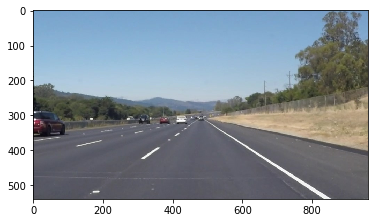Ideas for Lane Detection Pipeline¶

Some OpenCV functions (beyond those introduced in the lesson) that might be useful for this project are:

cv2.inRange() for color selection
cv2.fillPoly() for regions selection
cv2.line() to draw lines on an image given endpoints
cv2.addWeighted() to coadd / overlay two images cv2.cvtColor() to grayscale or change color cv2.imwrite() to output images to file
cv2.bitwise_and() to apply a mask to an image

Check out the OpenCV documentation to learn about these and discover even more awesome functionality!

Helper Functions¶

Below are some helper functions to help get you started. They should look familiar from the lesson!

In :
import math

def grayscale(img):
"""Applies the Grayscale transform
This will return an image with only one color channel
but NOTE: to see the returned image as grayscale
(assuming your grayscaled image is called 'gray')
you should call plt.imshow(gray, cmap='gray')"""
return cv2.cvtColor(img, cv2.COLOR_RGB2GRAY)
# return cv2.cvtColor(img, cv2.COLOR_BGR2GRAY)

def canny(img, low_threshold, high_threshold):
"""Applies the Canny transform"""
return cv2.Canny(img, low_threshold, high_threshold)

def gaussian_blur(img, kernel_size):
"""Applies a Gaussian Noise kernel"""
return cv2.GaussianBlur(img, (kernel_size, kernel_size), 0)

def region_of_interest(img, vertices):
"""

Only keeps the region of the image defined by the polygon
formed from vertices. The rest of the image is set to black.
"""

#defining a 3 channel or 1 channel color to fill the mask with depending on the input image
if len(img.shape) > 2:
channel_count = img.shape  # i.e. 3 or 4 depending on your image
else:

#filling pixels inside the polygon defined by "vertices" with the fill color

#returning the image only where mask pixels are nonzero

def draw_lines(img, lines, color=[255, 0, 0], thickness=2):
"""
NOTE: this is the function you might want to use as a starting point once you want to
average/extrapolate the line segments you detect to map out the full
extent of the lane (going from the result shown in raw-lines-example.mp4
to that shown in P1_example.mp4).

Think about things like separating line segments by their
slope ((y2-y1)/(x2-x1)) to decide which segments are part of the left
line vs. the right line.  Then, you can average the position of each of
the lines and extrapolate to the top and bottom of the lane.

This function draws lines with color and thickness.
Lines are drawn on the image inplace (mutates the image).
If you want to make the lines semi-transparent, think about combining
this function with the weighted_img() function below
"""
for line in lines:
for x1,y1,x2,y2 in line:
cv2.line(img, (x1, y1), (x2, y2), color, thickness)

def draw_avg_lines(img, lines, color=[255, 0, 0], thickness=2, info=dict()):
"""
This function draws two lines(left and right) by averaging line segments

Args:
img (np.array): image input where lines will be drawn
lines (list): [line1, line2, ...]
where line1 = (x1,y1, x2,y2)
which means a line segment
from pointA(x1, y1) to pointB(x2, y2)
color (list): [r, g, b] rgb value of color in list
thickness (int): thickness value
info (dict): store information to carry on
so if no line detected, we can keep assuming from the previous observation

"""

left_slope_data = info.get('left', [])[-50:]
right_slope_data = info.get('right', [])[-50:]

left_slope, left_bias = info.get('left_median', (0, 0))
right_slope, right_bias = info.get('right_median', (0, 0))

def get_slope(x1, y1, x2, y2):
"""Returns the slope and bias given two points
y = slope * x + bias
"""
slope = (y2 - y1) / (x2 - x1+1e-7)
bias = y1 - slope * x1
return slope, bias

def compute_avg_slope(slope_data, old_avg_slope, old_avg_bias, discount_rate = 0.5):

def get_running_avg(old, new, discount_rate):
if old == 0:
return new
return (1 - discount_rate) * old + discount_rate * new

if len(slope_data) > 0:
slope, bias = np.mean(slope_data, 0)
new_slope = get_running_avg(old_avg_slope, slope, discount_rate)
new_bias = get_running_avg(old_avg_bias, bias, discount_rate)

return new_slope, new_bias
return 0, 0

def draw_one_line(img, x, slope, bias):
point_a = (x, int(x * slope + bias))

point_b_y = img.shape // 100 * 68
point_b_x = int((point_b_y - bias) / (slope + 1e-7))

point_b = (point_b_x, point_b_y)

cv2.line(img, point_a, point_b, color, thickness)

return point_a, point_b

# if lines is not None
# for each line in lines
# get slope and bias from two given points in line
# if slope is < 0
# save to the left line
# else
# save to the right line
if lines is not None:
for line in lines:
for x1, y1, x2, y2 in line:
slope, bias = get_slope(x1, y1, x2, y2)
if slope < 0:
left_slope_data.append((slope, bias))
else:
right_slope_data.append((slope, bias))

# discount rate is used to compute an average on runtime
left_check = False
discount_rate = 0.9
left_slope, left_bias = compute_avg_slope(left_slope_data, left_slope, left_bias, discount_rate)
if left_slope != 0:
point_a, point_b = draw_one_line(img, 0, left_slope, left_bias)
left_check = True

right_check = False
right_slope, right_bias = compute_avg_slope(right_slope_data, right_slope, right_bias, discount_rate)
if right_slope != 0:
point_c, point_d = draw_one_line(img, img.shape, right_slope, right_bias)
right_check = True

poly_buffer = np.zeros_like(img)

if left_check and right_check:
cv2.fillPoly(poly_buffer, np.array([[point_a, point_c, point_d, point_b]], dtype=np.int32), [0, 255, 0])
img = cv2.addWeighted(poly_buffer, 0.1, img, 1.0, 0.)

info['left'] = left_slope_data
info['left_median'] = (left_slope, left_bias)
info['right'] = right_slope_data
info['right_median'] = (right_slope, right_bias)

return img

def hough_lines(img, rho, theta, threshold, min_line_len, max_line_gap, thickness=2, info=dict()):
"""
img should be the output of a Canny transform.

Returns an image with hough lines drawn.
"""
lines = cv2.HoughLinesP(img, rho, theta, threshold, np.array([]), minLineLength=min_line_len, maxLineGap=max_line_gap)
line_img = np.zeros((img.shape, img.shape, 3), dtype=np.uint8)

line_img = draw_avg_lines(line_img, lines, thickness=thickness, info=info)

return line_img

# Python 3 has support for cool math symbols.

def weighted_img(img, initial_img, α=0.8, β=1., λ=0.):
"""
img is the output of the hough_lines(), An image with lines drawn on it.
Should be a blank image (all black) with lines drawn on it.

initial_img should be the image before any processing.

The result image is computed as follows:

initial_img * α + img * β + λ
NOTE: initial_img and img must be the same shape!
"""
return cv2.addWeighted(initial_img, α, img, β, λ)

def color_select(img):
""" Select yellow and white portions of the image
"""
mask1 = cv2.inRange(img, np.array([150, 150, 0]), np.array([255, 255, 120]))
mask2 = cv2.inRange(img, np.array([220, 220, 220]), np.array([255, 255, 255]))
# img1 - yellow only img
# img2 - white only img

return cv2.bitwise_or(img1, img2)

Playground¶

• trying to search the best working pipeline
In :
temp = 'challenge.jpg'
ysize, xsize, _ = img.shape

# color select - yellow
temp = color_select(img)
mpimg.imsave("images/color_select.jpg", temp)
plt.imshow(temp, cmap='gray')
plt.show()

# gaussian blur - kernel 3

kernel_size = 9
temp = gaussian_blur(temp, kernel_size)
mpimg.imsave("images/gaussian_blur.jpg", temp)
plt.imshow(temp, cmap='gray')
plt.show()

# canny edge
low_threshold = 100
high_threshold = 255
temp = canny(temp, low_threshold, high_threshold)
cv2.imwrite("images/canny_edge.jpg", temp)
plt.imshow(temp, cmap='gray')
plt.show()

# ROI clipping
vertices = np.array([[(xsize//100*18, ysize), (xsize//100*99, ysize), (xsize//100*58, ysize//100*60), (xsize//100*50, ysize//100*60)]])
temp = region_of_interest(temp, vertices)
cv2.imwrite("images/roi_clipping.jpg", temp)
plt.imshow(temp, cmap='gray')
plt.show()

# Draw Hough lines
kwargs = {
'rho': 1,
'theta': np.pi/180*1,
'threshold': 50,
'min_line_len': 100,
'max_line_gap': 100,
}
temp = hough_lines(temp, **kwargs, thickness=10, info=dict())
plt.imshow(temp, cmap='gray')
plt.show()
mpimg.imsave("images/draw_hough_lines.jpg", temp)

# Mix with original image
temp = weighted_img(temp, img, 0.5, 1.0, 0.0)
plt.imshow(temp, cmap='gray')
plt.show()
mpimg.imsave("images/final_result.jpg", temp)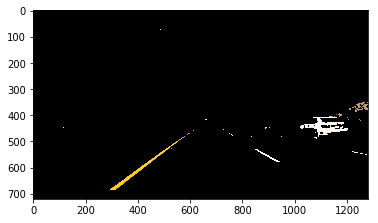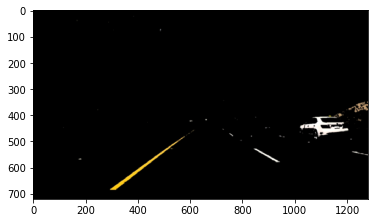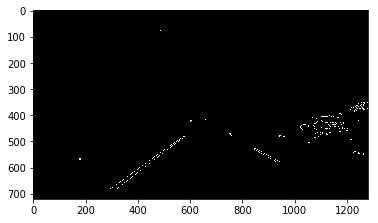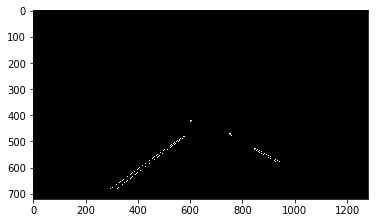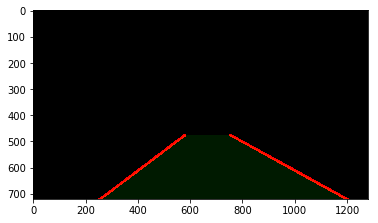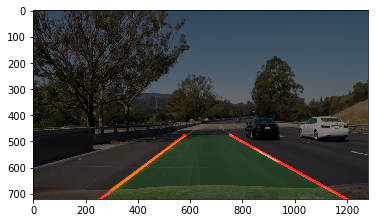Test Images¶

Build your pipeline to work on the images in the directory "test_images"
You should make sure your pipeline works well on these images before you try the videos.

In :
import os
os.listdir("test_images/")
Out:
['solidWhiteCurve.jpg',
'solidWhiteRight.jpg',
'solidYellowCurve.jpg',
'solidYellowCurve2.jpg',
'solidYellowLeft.jpg',
'whiteCarLaneSwitch.jpg']

Build a Lane Finding Pipeline¶

Build the pipeline and run your solution on all test_images. Make copies into the test_images directory, and you can use the images in your writeup report.

Try tuning the various parameters, especially the low and high Canny thresholds as well as the Hough lines parameters.

In :
def pipeline(img, info=dict()):
ysize, xsize, _ = img.shape #(540, 960, 3)
temp = color_select(img)
kernel_size = 9
blur = gaussian_blur(temp, kernel_size)
low_threshold = 50
high_threshold = 255
edge = canny(blur, low_threshold, high_threshold)
vertices = np.array([[(xsize//100*18, ysize), (xsize//100*99, ysize), (xsize//100*58, ysize//100*60), (xsize//100*50, ysize//100*60)]])
region = region_of_interest(edge, vertices)
kwargs = {
'rho': 2,
'theta': np.pi/180*1,
'threshold': 50,
'min_line_len': 100,
'max_line_gap': 200,
}
lines = hough_lines(region, **kwargs, thickness=5, info=info)
weighted = weighted_img(lines, img, 0.8)
return weighted

plt.figure(figsize=(15, 7))
for i, img in enumerate(os.listdir('test_images/')):
img_path = 'test_images/' + img
plt.subplot(2, 3, i+1)
info=dict()
plt.imshow(img_)
plt.title(img_path)
plt.show()
# then save them to the test_images directory.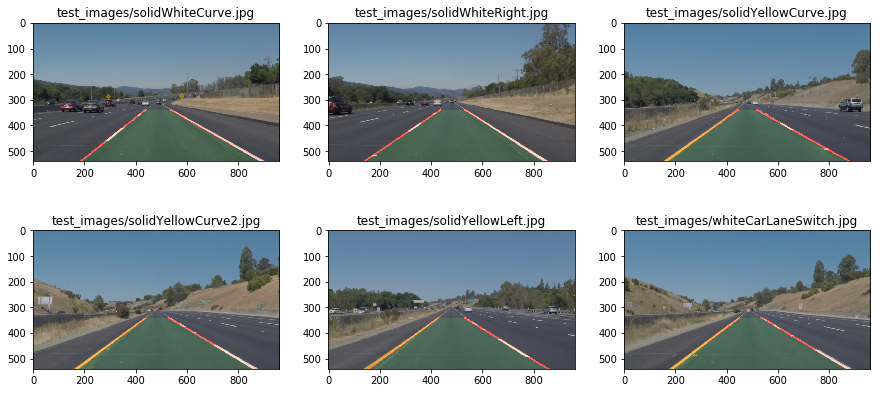Test on Videos¶

You know what's cooler than drawing lanes over images? Drawing lanes over video!

We can test our solution on two provided videos:

solidWhiteRight.mp4

solidYellowLeft.mp4

Note: if you get an import error when you run the next cell, try changing your kernel (select the Kernel menu above --> Change Kernel). Still have problems? Try relaunching Jupyter Notebook from the terminal prompt. Also, check out this forum post for more troubleshooting tips.

If you get an error that looks like this:

Follow the instructions in the error message and check out this forum post for more troubleshooting tips across operating systems.

In :
# Import everything needed to edit/save/watch video clips
from moviepy.editor import VideoFileClip
from IPython.display import HTML
In :
def process_image(image, info=dict()):
# NOTE: The output you return should be a color image (3 channel) for processing video below
# TODO: put your pipeline here,
# you should return the final output (image where lines are drawn on lanes)
result = pipeline(image, info=info)
return result

def process_helper(info):

def _process(img):
return process_image(img, info=info)

return _process

Let's try the one with the solid white lane on the right first ...

In :
white_output = 'white.mp4'
clip1 = VideoFileClip("solidWhiteRight.mp4")
ph = process_helper(dict())
white_clip = clip1.fl_image(ph) #NOTE: this function expects color images!!
%time white_clip.write_videofile(white_output, audio=False)
[MoviePy] >>>> Building video white.mp4
[MoviePy] Writing video white.mp4
100%|█████████▉| 221/222 [00:03<00:00, 60.18it/s]
[MoviePy] Done.

CPU times: user 4.54 s, sys: 970 ms, total: 5.51 s
Wall time: 4.03 s

Play the video inline, or if you prefer find the video in your filesystem (should be in the same directory) and play it in your video player of choice.

In :
HTML("""
<video width="960" height="540" controls>
<source src="{0}">
</video>
""".format(white_output))
Out:

Improve the draw_lines() function¶

At this point, if you were successful with making the pipeline and tuning parameters, you probably have the Hough line segments drawn onto the road, but what about identifying the full extent of the lane and marking it clearly as in the example video (P1_example.mp4)? Think about defining a line to run the full length of the visible lane based on the line segments you identified with the Hough Transform. As mentioned previously, try to average and/or extrapolate the line segments you've detected to map out the full extent of the lane lines. You can see an example of the result you're going for in the video "P1_example.mp4".

Go back and modify your draw_lines function accordingly and try re-running your pipeline. The new output should draw a single, solid line over the left lane line and a single, solid line over the right lane line. The lines should start from the bottom of the image and extend out to the top of the region of interest.

Now for the one with the solid yellow lane on the left. This one's more tricky!

In :
yellow_output = 'yellow.mp4'
clip2 = VideoFileClip('solidYellowLeft.mp4')
ph = process_helper(dict())
yellow_clip = clip2.fl_image(ph)
%time yellow_clip.write_videofile(yellow_output, audio=False)
[MoviePy] >>>> Building video yellow.mp4
[MoviePy] Writing video yellow.mp4
100%|█████████▉| 681/682 [00:11<00:00, 60.55it/s]
[MoviePy] Done.

CPU times: user 13.9 s, sys: 2.73 s, total: 16.6 s
Wall time: 11.6 s
In :
HTML("""
<video width="960" height="540" controls>
<source src="{0}">
</video>
""".format(yellow_output))
Out:

Writeup and Submission¶

If you're satisfied with your video outputs, it's time to make the report writeup in a pdf or markdown file. Once you have this Ipython notebook ready along with the writeup, it's time to submit for review! Here is a link to the writeup template file.

Optional Challenge¶

Try your lane finding pipeline on the video below. Does it still work? Can you figure out a way to make it more robust? If you're up for the challenge, modify your pipeline so it works with this video and submit it along with the rest of your project!

In :
challenge_output = 'extra.mp4'
clip2 = VideoFileClip('challenge.mp4')
ph = process_helper(dict())
challenge_clip = clip2.fl_image(ph)
%time challenge_clip.write_videofile(challenge_output, audio=False)
[MoviePy] >>>> Building video extra.mp4
[MoviePy] Writing video extra.mp4
100%|██████████| 251/251 [00:08<00:00, 28.25it/s]
[MoviePy] Done.